Function Repository Resource:

# QuantileOperator

An operator form of the Quantile function

Contributed by: Seth J. Chandler
 ResourceFunction["QuantileOperator"][q] gives an operator for retrieving the qth quantile from data. ResourceFunction["QuantileOperator"][q,{{a,b},{c,d}}] uses the quantile definition specified by parameters a,b,c,d.

## Details and Options

The data can be a List or statistical distribution.
The value of q should be between zero and one.
The effect of the various parameters is detailed in the documentation for the Quantile function.

## Examples

### Basic Examples (2)

Create an operator that will find the halfway-value (median) of a List or statistical distribution:

 In:=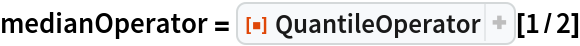Out=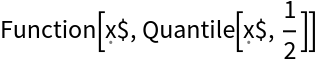Apply the operator to a List and a distribution:

 In:=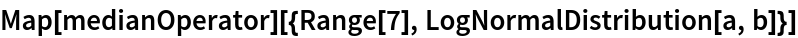Out=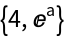Create an operator that computes lower and upper quartiles for whatever data it encounters, and then map it over multiple sets of data:

 In:=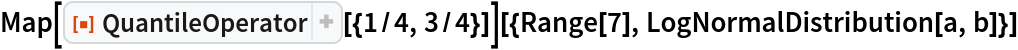Out=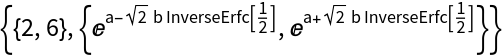### Scope (2)

Create an Association in which the keys are various parameters to the Quantile function and the values are each a corresponding QuantileOperator:

 In:=Out=Apply the different QuantileOperator expressions to the same data to see how the choice of parameters affects the outcome:

 In:=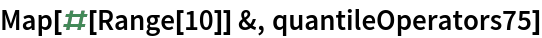Out=### Applications (2)

Make a Histogram of the lower and upper quartiles of 100 random draws (10,000 samples) from a normal distribution:

 In:=Out=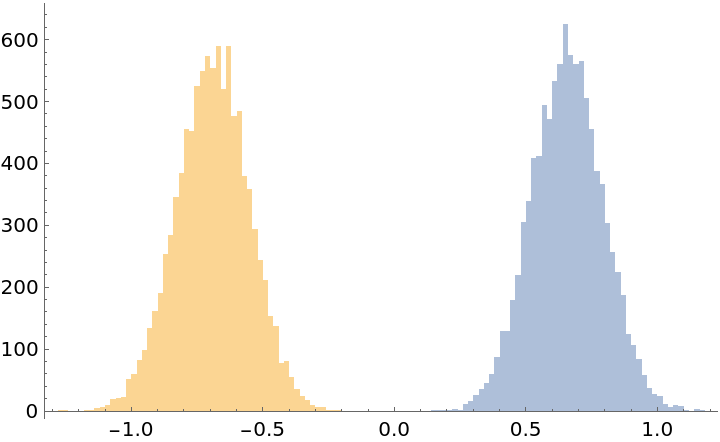Use quantile as a mesh function in drawing the PDF of a normal distribution:

 In:=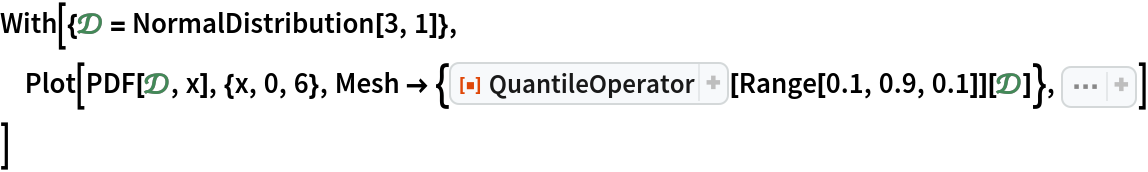Out=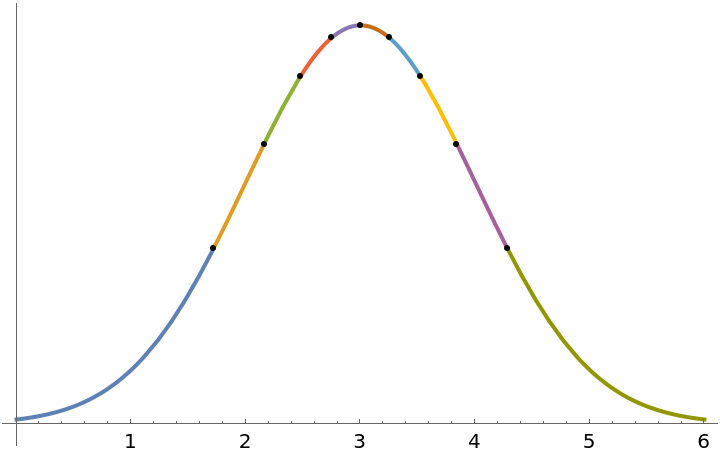### Properties and Relations (2)

QuantileOperator when used with a single argument is equivalent (as of version 12.0) to :

 In:=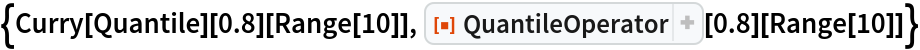Out=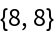QuantileOperator[0.5,{{1/3,1/3},{0,1}}] is equivalent to Median:

 In:=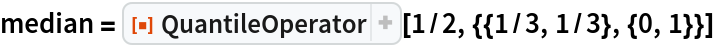Out=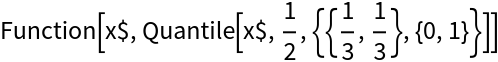In:=Out=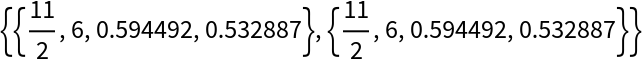Seth J. Chandler

## Version History

• 1.0.0 – 17 December 2019